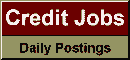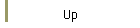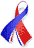DefaultRisk.com the web's biggest credit risk modeling resource.doi> search: A or B Export citation to:- HTML- Text (plain)- BibTeX- RIS- ReDIF

Efficient Pricing Routines of Credit Default Swaps in a Structural Default Model with Jumps

by Matthias Scherer of the University of Ulm

December 2, 2005

Abstract: In this paper, we present two efficient algorithms for pricing credit default swaps based on a structural default model. In our model, the value of the firm is assumed to be the exponential of a jump-diffusion process. Our first algorithm to price a credit default swap within this framework is an efficient and unbiased Monte Carlo simulation. An excellent performance is obtained by first simulating the jump component and then analytically evaluating the pricing formula conditioned on the simulated jumps. This technique is flexible enough to allow all possible jump distributions. The second approach uses the Laplace transform of first-passage times. This transform is analytically tractable for a jump-diffusion process with two-sided exponentially distributed jumps. Combined with the Gavard-Stehfest algorithm for Laplace inversion, this provides a very fast method for finding default probabilities based on which credit default swap prices can be computed. Within the framework of a jump diffusion with two-sided exponentially distributed jumps, we present a sensitive analysis of the parameters and compute the exact limit of resulting par spreads at the short end of the term structure. In contrast to pure diffusion models, this term structure of par spreads has a non-zero limit that matches empirical observations.

Books Referenced in this paper:  (what is this?)